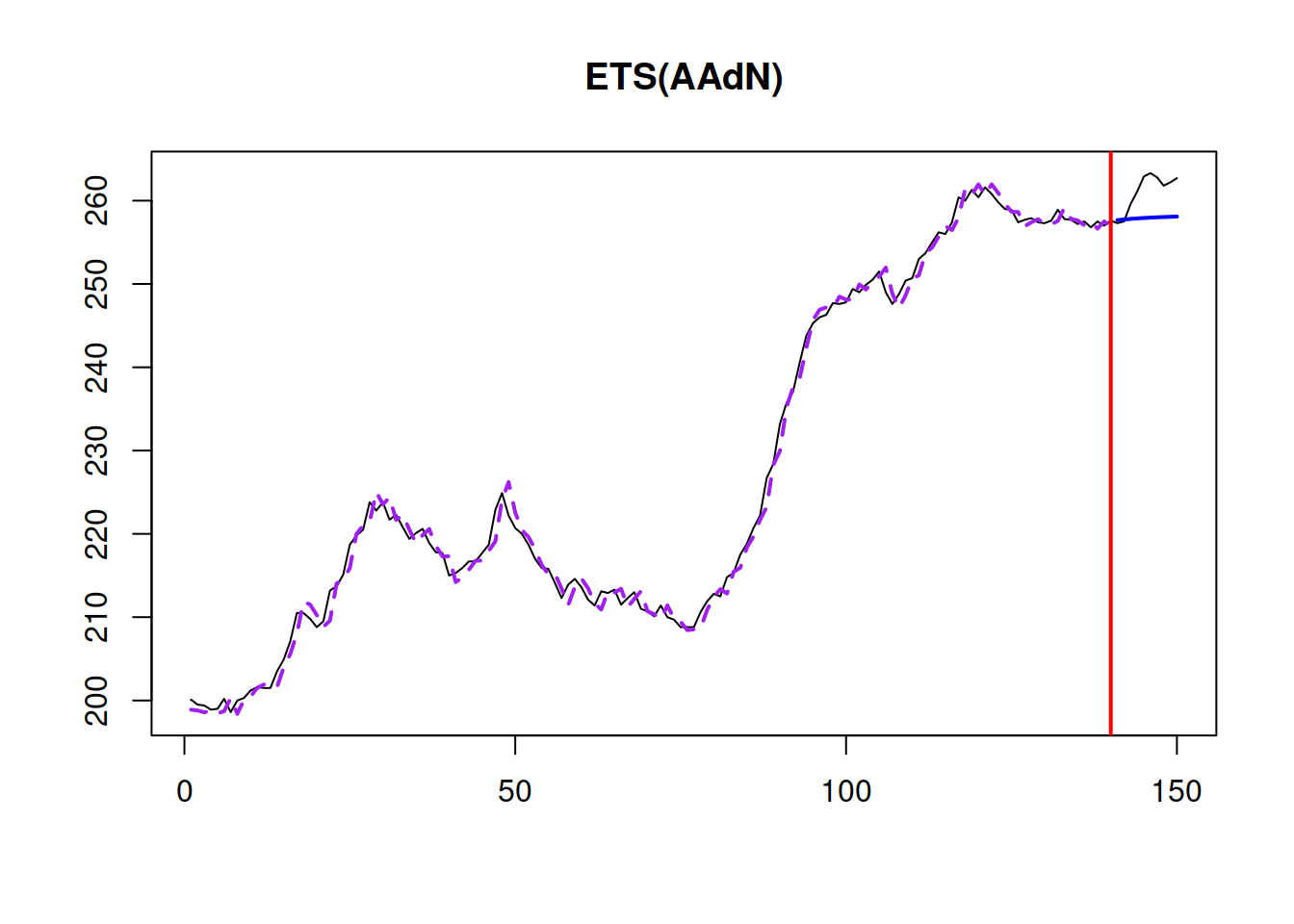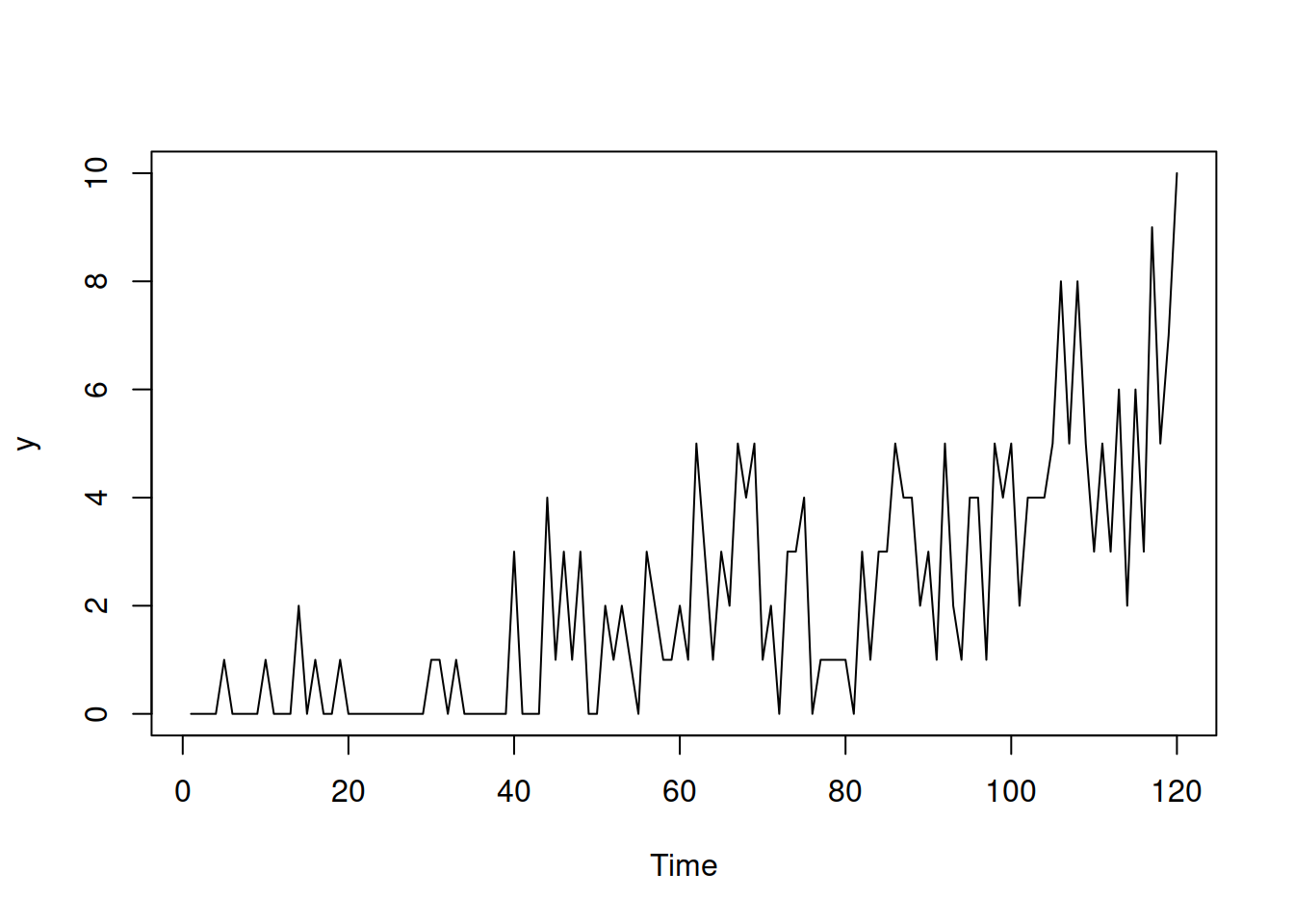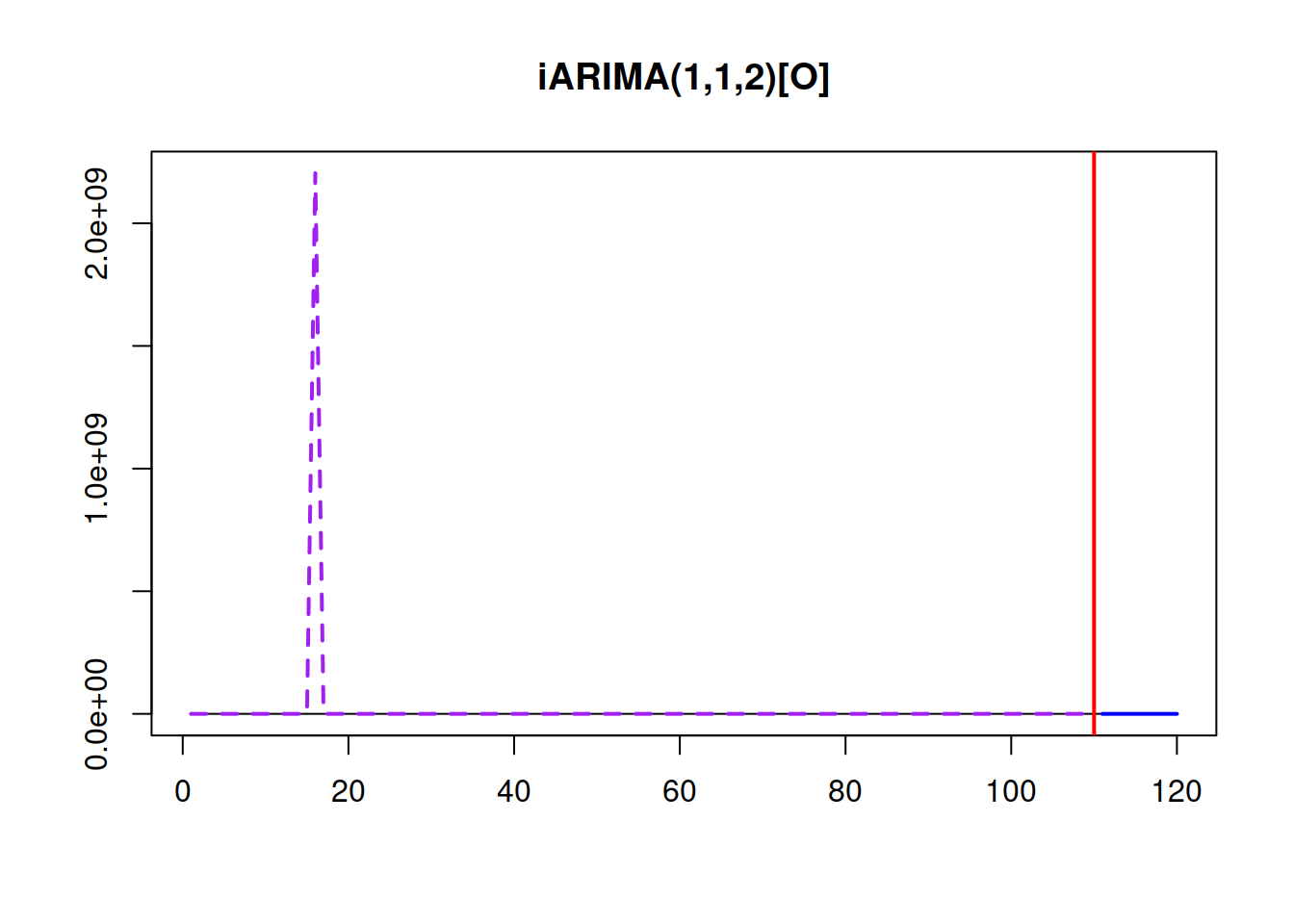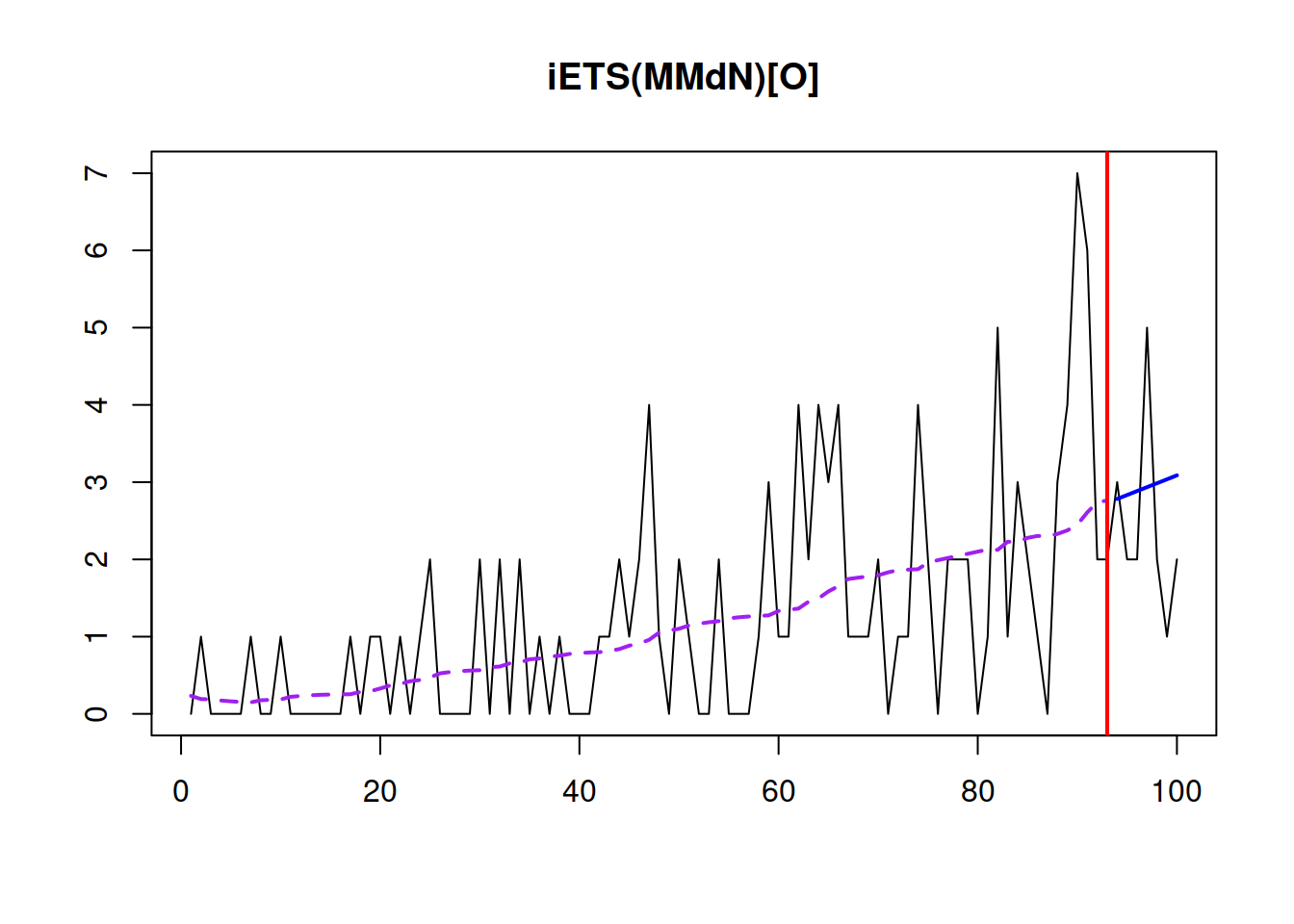This book is in Open Review. I want your feedback to make the book better for you and other readers. To add your annotation, select some text and then click the on the pop-up menu. To see the annotations of others, click the button in the upper right hand corner of the page

## 17.8 Residuals are i.i.d.: distributional assumptions

Finally, we come to the distributional assumptions of ADAM. As discussed earlier (for example, in Section 14.1), ADAM framework supports several distributions, and the specific parts of assumptions will change depending on the type of the error term in the model. Given that, it is relatively straightforward to see if the residuals of the model follow the assumed distribution or not. There exist several tools for that.

The simplest one is called Quantile-Quantile (QQ) plot. It produces a figure with theoretical vs actual quantiles and shows, whether they are close to each other or not. Here is, for example, how the QQ plot will look for one of the previous models, assuming Normal distribution:

plot(adamModelSeat03,6)If the residuals do not contradict the assumed distribution, then all the points should lie either very close to or on the line. In our case, the majority of points are close to the line, but the tails are slightly off. In ADAM, this might mean that we should either use a different error type or a different distribution. Just for the sake of argument, we can try ETSX(M,N,M) model, with the same set of explanatory variables as in the model adamModelSeat03, and with the same Normal distribution:

adamModelSeat16 <- adam(Seatbelts,"MNM",formula=drivers~PetrolPrice+kms+front+rear+law,
distribution="dnorm")
plot(adamModelSeat16,6)According to the new QQ plot, the residuals of the new model are much closer to the theoretical ones, there is now only the right tail that is wrong - the actual values are a bit further away than expected. This can be addressed by using a skewed distribution, for example, Inverse Gaussian:

adamModelSeat17 <- adam(Seatbelts,"MNM",formula=drivers~PetrolPrice+kms+front+rear+law,
distribution="dinvgauss")
plot(adamModelSeat17,6)The new QQ plot demonstrates that the empirical residuals follow the assumed distribution much closer than in the previous cases: there are just few observations that lie slightly away from the line, but they could happen at random. So, based on this simple analysis we could conclude that Inverse Gaussian distribution is more suitable for this situation than the Normal one. Interestingly, this is supported by the AIC values, which very roughly reflect the same thing:

setNames(c(AIC(adamModelSeat03),AIC(adamModelSeat16),AIC(adamModelSeat17)),
c("Additive Normal","Multiplicative Normal","Multiplicative IG"))
##       Additive Normal Multiplicative Normal     Multiplicative IG
##              2228.579              2219.542              2232.435

Another way to analyse the distribution of residuals is to plot histogram together with the theoretical density function. Here is an example:

hist(residuals(adamModelSeat03), probability=TRUE)
lines(seq(-250,250,1),
dnorm(seq(-250,250,1), 0, sd(residuals(adamModelSeat03))),
col="red")However, this plot is much more difficult to analyse than QQ plot, because of the bars, which average out the quantiles. So, in general I would recommend using QQ plots instead.

There are also formal tests for the distribution of residuals, such as Shapiro-Wilk (Wikipedia, 2021h) and Kolmogorov-Smirnov (Wikipedia, 2021i). The former tests the hypothesis that residuals follow Normal distribution, while the latter one is much more flexible and allows comparing the empirical distribution with any other (theoretical or empirical). However, I prefer to use visual inspection, when possible instead of doing these tests because, as we discussed earlier in Section 2.5, the null hypothesis is always wrong, and it will inevitably be rejected with the increase of the sample size. Besides, if you fail to reject H$$_0$$, it does not mean that your variable follows the assumed distribution, it only means that you have not found enough evidence to reject it.

### References

• Wikipedia, 2021h. Shapiro-Wilk test. https://en.wikipedia.org/wiki/Shapiro%E2%80%93Wilk_test (version: 2021-06-09)

• Wikipedia, 2021i. Kolmogorov-Smirnov test. https://en.wikipedia.org/wiki/Kolmogorov%E2%80%93Smirnov_test (version: 2021-06-09)